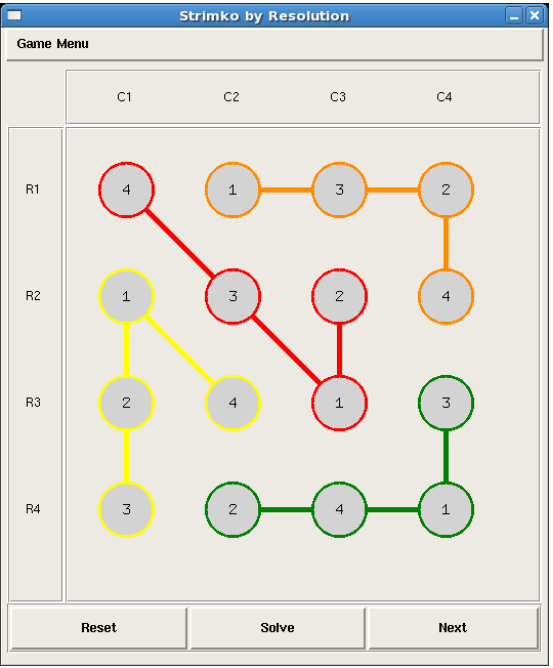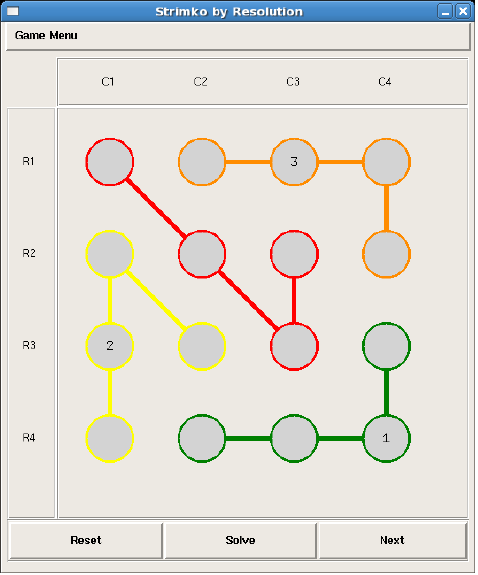This project led to a publication in the conference journal of the Association for the Advancement of Artificial Intelligence (AAAI) 2014. This project was designed as an undergraduate classroom project in artificial intelligence. I worked on this assignment in coordination with my advisor. The project uses the game Strimko which is similar to the popular Sudoku.

In Strimko, you have grid of circles, but the circles are also connected to each other. Like Sudoku, the grid must contain all the numbers from 1 through the max size, so for a 4x4 grid each row and column must contain 1,2,3, and 4 in some order. However, in Strimko, instead of the grids containing squares that must also contain the numbers, it contains chains that stretch all over the board. These chains must also contain the numbers in some order. As can be seen in the below image, each column, row, and chain contain some ordering of 1 through 4.To create the solver, the puzzle is represented as propositional logic. Propositional logic is generated as follows.

We define $$c_{i,j}^k$$ as cell (i,j) contains the number k. So, in the above picture, $$c_{1,1}^4$$ is true because (R1,C1) has a value of 4.

We can combine these together into disjunctions for each row, column, and chain. Which will give a form that looks like $$c_{i,1}^{k} \lor c_{i,2}^{k} \lor c_{i,3}^{k} \lor ... \lor c_{i,n}^{k}$$. This disjunction shows that the number k must be somewhere in row i.

The second disjunction type will be for each cell (i,j) and will look like $$c_{i,j}^{1} \lor c_{i,j}^{2} \lor ... \lor c_{i,j}^{n}$$ which means that each cell must be filled with some number 1 through n.

These four sets of disjunctions, will be out list of starting facts. Once a number has been chosen, i.e when $$c_{ij}^{k} = true$$, we know (i,j) must contain k. Since a correct number is known, we can add a new conjunction of the form $$\neg c_{i,1}^{k} \land \neg c_{i,2}^{k} \land ... \land \neg c_{i,j-1}^{k} \land ... \land \neg c_{i,n}^{k}$$ which means that the number k can only occur once in the entire row. This same conjunction can be created for the columns and chains.

The final proposition is related to the previous, it states that cell (i,j) may contain nothing other than k. It takes the form $$\neg c_{i,j}^{1} \land \neg c_{i,j}^{2} \land ... \land \neg c_{i,j}^{k-1} \land \neg c_{i,j}^{k+1} \land ... \land \neg c_{i,j}^{n}$$Since this is used as a classroom assignment, I can’t go into too much detail of the implentation, but I can make a brief example of the logic behind how this works. Take the above image for example.

Given the red chain, it will have a disjunction of $$c_{1,1}^{3} \lor c_{2,2}^{3} \lor c_{3,3}^{3} \lor c_{2,3}^{4}$$ which says that the number 3 must appear somewhere in the red chain. Now, notice cell (R1,C3) has the value of 3. This means that the remaining cells in row R1 and column C2 cannot contain a 3. Once the algorithm processes that evidence, it will remove $$c_{1,1}^{3}$$, $$c_{3,3}^{3}$$, and $$c_{2,3}^{3}$$ from the disjunction since 3 is already in R1 and C2. This leaves only a disjunction of $$c_{2,2}^{3}$$ which means the value for cell (R2,C2) must be 3.

So, in order to fully solve the puzzle, you repeat these steps. You have a piece of evidence that is true, and you loop over all the disjunctions and remove any contradictions of that evidence. If the puzzle is solveable, after each iteration, there should be at least one disjunction with a single value left. That value is the solution to a cell. That value is assigned to the cell, and the process is repeated. This new cell should give the algorithm the ability to remove more contradictions until the puzzle is solved.

This assignment was published in the AAAI 14 conference journal. I do not have a direct copy of the journal, but I have the posted abstracts, the assignment pdf, and the classroom assignment code. The classroom code has all of the graphical backend but is missing the propositional logic code since the students are required to write that for the assignment. I will link all below.

Strimko Assignment

The Strimko assignment is listed on my Github.